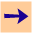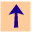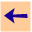4 Ask and Tell

The general interaction with CILOG is to tell it clauses and to ask it queries (which are bodies). It replies with answers, which are instances of the query that are consequences of its knowledge base. The user can then either explore how the system derived an answer or why the system didn't find another answer.

To add a clause to the knowledge base you issue the command:

```cilog: tell clause.
```

Example. You could tell the system some genealogy knowledge:

```cilog: tell parent(X,Y) <- mother(X,Y).
cilog: tell parent(X,Y) <- father(X,Y).
cilog: tell grandfather(X,Y) <- father(X,Z) & parent(Z,Y).
cilog: tell grandmother(X,Y) <- mother(X,Z) & parent(Z,Y).
cilog: tell father(randy,sally).
cilog: tell father(randy,joe).
cilog: tell mother(sally,mary).
cilog: tell father(joe,sue).
```

You can ask a query to determine if some expression is a consequence of the knowledge base:
```cilog: ask query.
```
where query is an expression. The system will respond with either an instance of the query that is a consequence of the knowledge base or "No. query doesn't follow from the knowledge base" if there are no answers. When the system have given an answer you can reply with:
ok.
to indicate that you don't want any more answers
more.
how.
to investigate how that answer was produced (see Section 6.1)
help.
to get a menu of available answers (this option is available at all points where the system is asking for a response)
If you reply with more, the system either finds another answer or reports there are no more answers.

Example. Given the knowledge base after Example 4, you can ask queries of the knowledge base:

```cilog: ask grandfather(randy,mary).
[ok,more,how,help]: ok.
```
It has thus told you that grandfather(randy,mary) is a consequence of the knowledge base.
```cilog: ask grandfather(joe,mary).
No. grandfather(joe,mary) doesn't follow from the knowledge base.
```
which means grandfather(joe,mary) is not a consequence of the knowledge base.

You can ask queries with free variables:

```cilog: ask grandfather(G,C).4 Ask and Tell